To thousands

Round to thousands following numbers:

Result

274 538:  275000
774 186:  774000
16 267:  16000
969 562:  970000
946 605:  947000
354 780:  355000
909 842:  910000
560 471:  560000
202 360:  202000
592 802:  593000

Leave us a comment of example and its solution (i.e. if it is still somewhat unclear...):Be the first to comment!Next similar examples:

1. Rounding 2Round the following negative number: -143.021
2. Valid numberRound the 453874528 on 2 significant numbers.
3. Rounding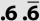Double round number 727, first to tens, then to hundreds. (double rounding)
4. Sum and roundingI know two numbers whose sum is 20. When they each round and then added together I get the 30. What are this numbers?
5. SavingMom said that Suzan save about 120 EUR (rounded to tens). How many euros she could at least save (minimum)?
6. Like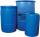When is in 14 barrels 140 liters of water, how many liters barrels of 8 liters I need to get all the water from the larger barrels?
7. Roman numerals 2+Add up the number writtens in Roman numerals. Write the results as a roman numbers.
8. ProductResult of the product of the numbers 1, 2, 3, 1, 2, 0 is:
9. Roman numeralsWrite numbers written in Roman numerals as decimal.Added together and write as decimal number: LXVII + MLXIV
11. Roman numerals +Add up the number writtens in Roman numerals. Write the results as a decimal number.
12. Compare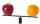Compare with characters >, <, =: 85.57 ? 80.83
13. DoctorsIn the city operates 171 doctors. The city has 128934 citizens. How many citizens are per one doctor?
14. Baking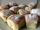There are 28 bunches, and son ate 1/2, dad ate four bunches. How many of them remain on the baking dishes?
15. Book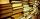Jitka read on holidays book that has 180 pages. In the first week read 45 pages. In the second week she read 15 pages more than the first week. How many pages left to read it yet?
16. Train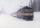The train passes part of the line for 95 minutes at speed 75 km/h. What speed would have to go in order to shorten the driving time of 20 minutes?
17. TriangleProve whether you can construct a triangle ABC, if a=9 cm, b=10 cm, c=4 cm.